﻿• 价格透明
• 信息保密
• 进度掌控
• 售后无忧# 1，条件查询

``````select  字段1，字段2... from 表名 where 条件1，条件2...
``````

## 1.1 条件运算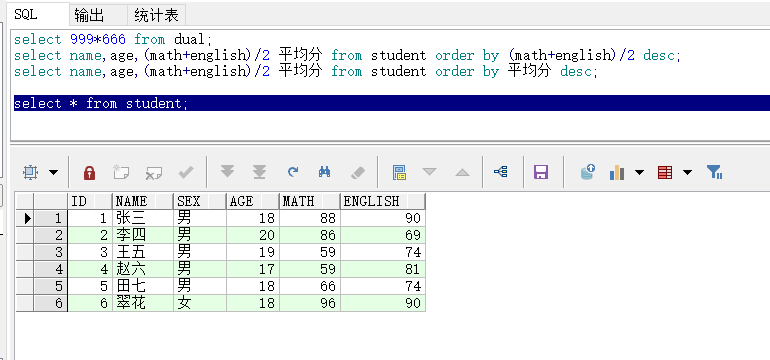``````select * from student where sex = '女';
``````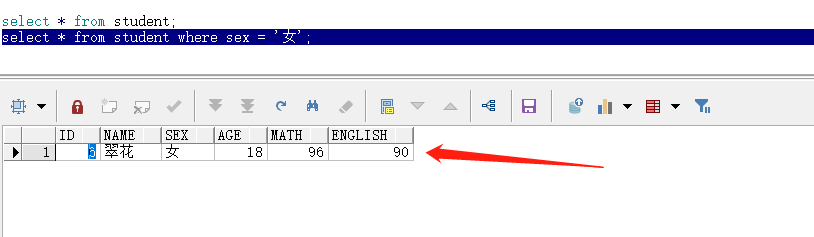``````select * from student where english > 80;
``````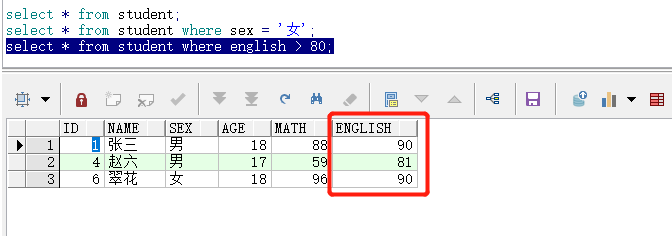``````select * from student where math between 60 and 90;
``````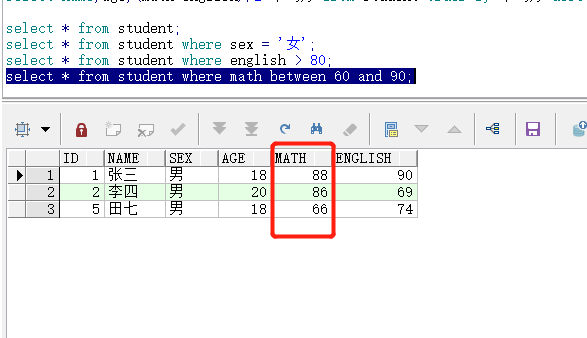``````select * from student where age in (18,20);
``````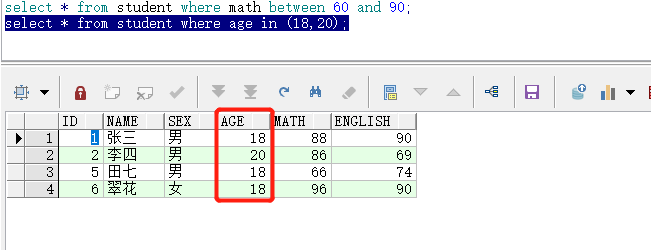`in`后面括号里放有限个数值，并不是表示区间。

`<>`表示不等于，以及`^=``!=`也是不等于.

## 1.2 条件连接运算

`and`示例：查询数学成绩大于`60`并且英语成绩大于`80`的所有记录：

``````select * from student where math > 60 and english > 80;
``````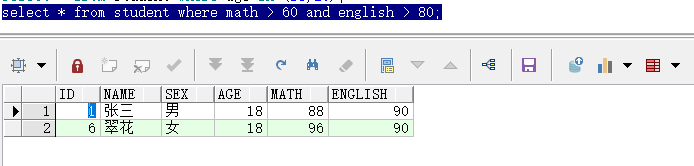`or`实例：查询年龄大于`19`或者英语成绩小于`80`的所有记录：

``````select * from student where age > 19 or english < 80;
``````

`or`表示只要满足其中一个条件，就会被查询出来。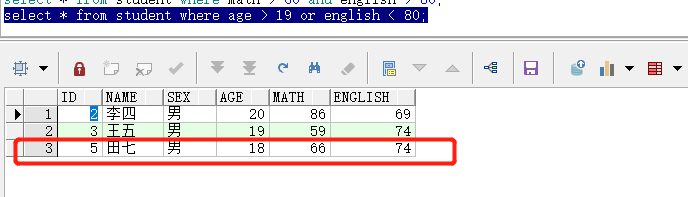`not`示例：查询`sex`为女的所有记录：

``````select * from student where not sex = '男';
``````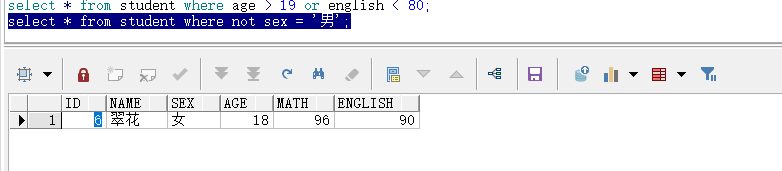## 1.3 null运算nvl()

`is null , is not null , not ... is null`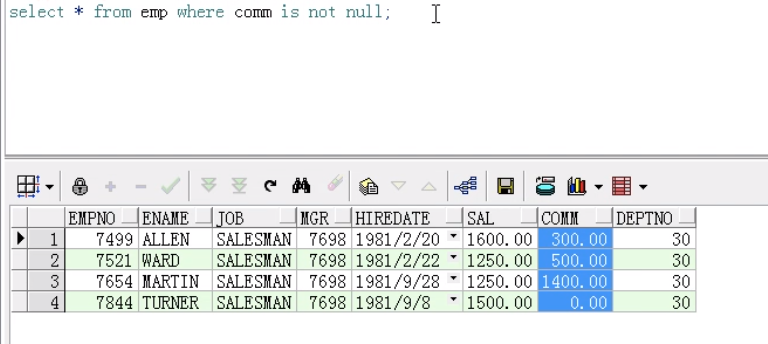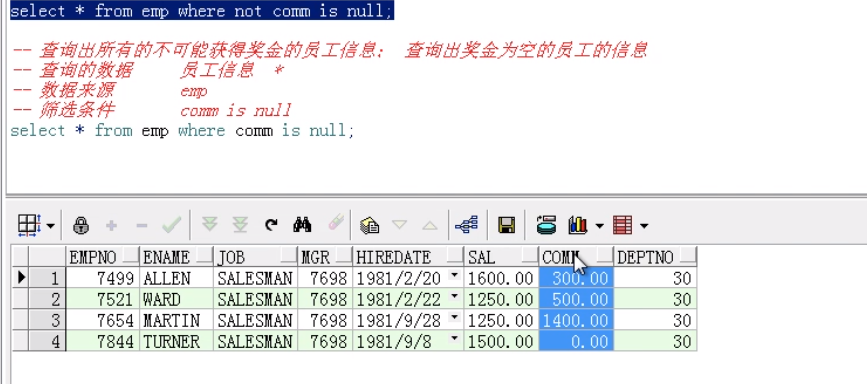## 1.4 模糊查询 like

• `%` 表示占位符，可以占多个位置
• `_`表示占位符，只能占一位
例如：查询名字你们带`王`字的
``````select * from student where name like '%王%';
``````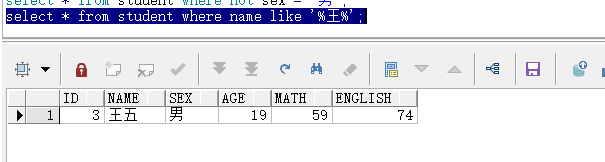``````select * from student where name like '张_';
``````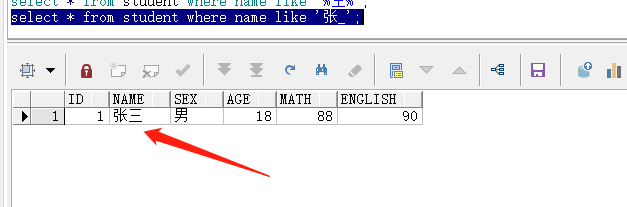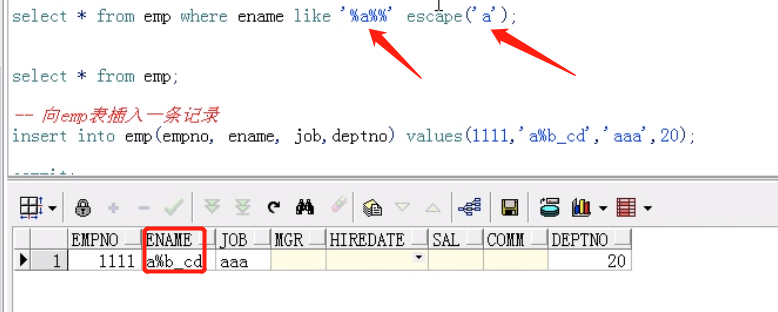### 低价透明### 金牌服务### 信息保密